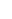# A particle moves along the x axis from x = 12.8 m to x = 23.7 m under the influence of a force F = 375 x 3 + 3.75 x where F is in newtons and x is in meters. Using numerical integration, determine the work done by this force on the particle during this displacement. Your result should he accurate to within 2%.### Physics for Scientists and Enginee...

10th Edition
Raymond A. Serway + 1 other
Publisher: Cengage Learning
ISBN: 9781337553278### Physics for Scientists and Enginee...

10th Edition
Raymond A. Serway + 1 other
Publisher: Cengage Learning
ISBN: 9781337553278

#### Solutions

Chapter
Section
Chapter 7, Problem 43AP
Textbook Problem
1050 views

## A particle moves along the xaxis from x = 12.8 m to x = 23.7 m under the influence of a force F = 375 x 3 + 3.75 xwhere F is in newtons and x is in meters. Using numerical integration, determine the work done by this force on the particle during this displacement. Your result should he accurate to within 2%.

Expert Solution
To determine

The work done by the force during the displacement of the particle.

### Explanation of Solution

The force acting on the particle is F=375x3+3.75x and the particle moves along the x-axis from x=12.8m to x=23.7m.

Write the formula to calculate the work done by the force

W=xixfFdx

Here, F is the force acting on the particle, xf is the final position of the particle and xi is the initial position of the particle.

Conclusion:

Substitute 375x3+3.75x for F, 12.8m for xi and 23.7m for xf to find W.

W=[12.823.7(375x3+3

### Want to see the full answer?

Check out a sample textbook solution.See solution

### Want to see this answer and more?

Bartleby provides explanations to thousands of textbook problems written by our experts, many with advanced degrees!

See solution